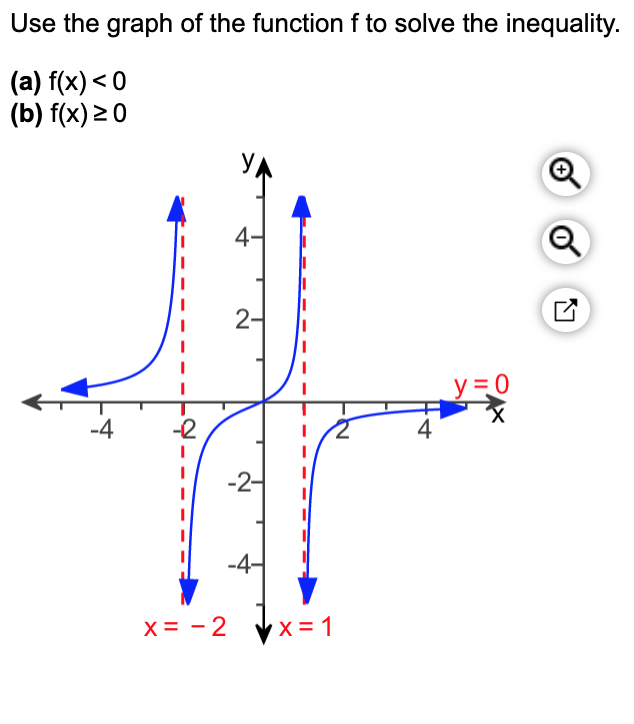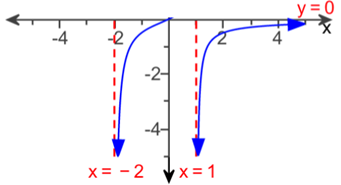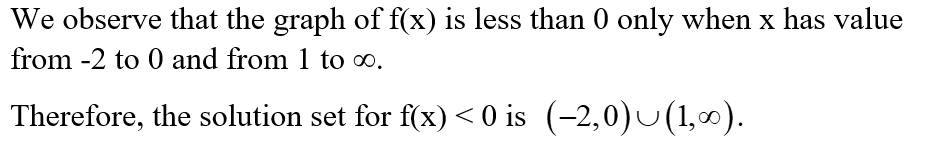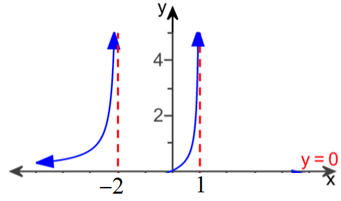# Use the graph of the function f to solve the inequality.(a) f(x) < 0(b) f(x) 20У4-2-У 3D0-2-4-2--4-X = - 24-

Question
63 views
Use the graph of the function f to solve the inequality.

​(a) f(x)less than<0
​(b) f(x)greater than or equals≥0

x=-2
x=1
y=0help_outlineImage TranscriptioncloseUse the graph of the function f to solve the inequality. (a) f(x) < 0 (b) f(x) 20 У 4- 2- У 3D0 -2 -4 -2- -4- X = - 2 4- fullscreen
check_circle

Step 1

(a) f(x) < 0

Consider the graph of f(x) for f(x) < 0.Step 2Step 3

(b) f(x) ≥ 0

Now consider the graph of f(x) for f(x) ≥ 0....

### Want to see the full answer?

See Solution

#### Want to see this answer and more?

Solutions are written by subject experts who are available 24/7. Questions are typically answered within 1 hour.*

See Solution
*Response times may vary by subject and question.
Tagged in